This video explains how to find the equation of a line when given 2 points.

Find the slope using the slope formula,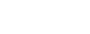$m = \dfrac{y_2 - y_1}{x_2 - x_1}$.
After finding the slope, plug in the value of your points into either slope-intercept form, or point-slope form, to get the equation of the line. After you finish this lesson, view all of our Algebra 1 lessons and practice problems.

## Examples of Finding The Equation Of A Line, Given Two Points

### Example 1

Find the equation of the line passing through the points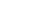$(-5, 7)$ and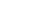$(2, 3)$.

First, let’s find the slope.

Let’s label the given: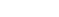$x_1 = -5$,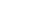$y_1 = 7$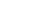$x_2 = 2$,$y_2 = 3$$m = \dfrac{y_2 - y_1}{x_2 - x_1}$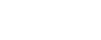$m = \dfrac{3 - 7}{2 - (-5)}$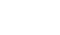$m = \dfrac{-4}{7}$

Next, let’s find the y-intrecept by using slope intercept form.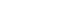$y = mx + b$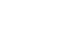$y = \dfrac{-4}{7}x + b$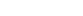$7y=-4x+7b$

Substitute any of the paired coordinates but not both.$x_1 = -5$,$y_1 = 7$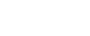$7(7) = \dfrac{-7} {-5}+ 7b$

Let’s solve for$b$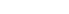$49 = 20 + 7b$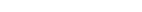$49- 20 = 20 +7b-20$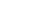$29 = 7b$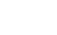$\dfrac{29}{7} = \dfrac{7b}{7}$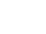$\dfrac{29}{7} = b$

Now, we know what the y-intercept is and the slope.
So the equation$y = mx + b$ becomes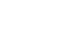$y = \dfrac{-4}{7}x + \dfrac{29}{7}$

### Example 2

Find the equation of the line passing through the points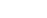$(-4, 2)$ and$(1, -6)$

First, let’s find the slope.

Let’s label the given: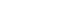$x_1 = -4$,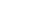$y_1 = 2$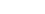$x_2 = 1$,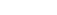$y_2 = -6$$m = \dfrac{y_2 - y_1}{x_2 - x_1}$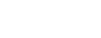$m = \dfrac{-6 - 2}{1- (-4)}$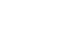$m = \dfrac{-8}{5}$

Next, let’s solve using point slope form.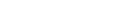$y - y_1 = m (x - x_1)$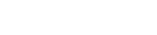$y - 2 = \dfrac{-8}{5} (x - (-4))$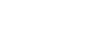$y - 2 = \dfrac{-8}{5} (x +4)$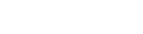$y - 2 = \dfrac{-8}{5}x -\dfrac{8}{5}(4)$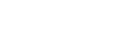$y - 2 = \dfrac{-8}{5}x -\dfrac{32}{5}$

Now, let’s get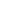$y$ by itself by adding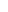$2$ on both sides.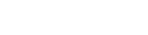$y - 2 +2 = \dfrac{-8}{5}x -\dfrac{32}{5}+2$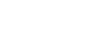$y = \dfrac{-8}{5}x -\dfrac{22}{5}$

## Video-Lesson Transcript

Let’s find an equation of a line, given two points.

How do we do this?

We can solve this by using the slope intercept form:$y = mx + b$

or by using point-slope formula:$y - y_1 = m (x - x_1)$

But in both cases we need to know what the slope (m) is.

So the first step is to find the slope.

The formula for finding the slope is$m = \dfrac{y_2 - y_1}{x_2 - x_1}$

For example:

Find the equation of a line with given points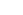$2, 4$ and$4, 5$

First, let’s find the slope.

Let’s label the given: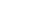$x_1 = 2$,$y_1 = 4$$x_2 = 4$,$y_2 = 5$$m = \dfrac{y_2 - y_1}{x_2 - x_1}$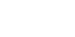$m = \dfrac{5 - 4}{4 - 2}$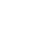$m = \dfrac{1}{2}$

So now, we can find the equation of a line using the slope intercept form or the point slope form.

Let’s do the slope intercept form first.$y = mx + b$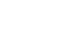$y = \dfrac{1}{2}x + b$

Now, you can substitute any of the paired coordinates but not both.

I’m going to use the second pair of coordinates$x_2 = 4$,$y_2 = 5$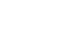$5 = \dfrac{1}{2} (4) + b$

Now, let’s solve for$b$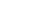$5 = 2 + b$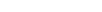$5 - 2 = 2 - 2 + b$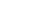$3 = b$

Now, we know what the y-intercept is and the slope.

So the equation$y = mx + b$ becomes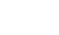$y = \dfrac{1}{2}x + 3$Now, let’s solve using the point-slope form.$y - y_1 = m (x - x_1)$

Here, we can choose which pair to use as coordinates.

So let me choose the first set of coordinates$x_1 = 2$,$y_1 = 4$

Also, we already know what the slope is$m = \dfrac{1}{2}$

Now, let’s solve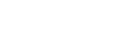$y - 4 = \dfrac{1}{2} (x - 2)$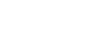$y - 4 = \dfrac{1}{2}x - 1$

Let’s get$y$ by itself by adding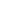$4$ on both sides.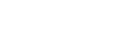$y - 4 + 4 = \dfrac{1}{2}x - 1 + 4$$y = \dfrac{1}{2}x + 3$